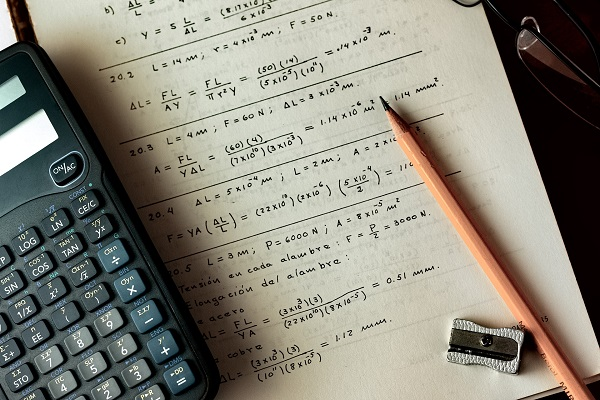# What Is the Difference Between Pure Math and Applied Math?

A master’s in applied mathematics, one of the highest-paying master’s degrees, isn’t your only option for graduate study in math. Applied math is a different area of study and professional practice than pure math. While work in pure math explores mathematical knowledge for its own sake, work in applied math emphasizes the use of mathematical principles for practical purposes. There are significant differences in the curricula across these two math-focused programs of study.

DegreeQuery.com is an advertising-supported site. Featured or trusted partner programs and all school search, finder, or match results are for schools that compensate us. This compensation does not influence our school rankings, resource guides, or other editorially-independent information published on this site.

## Math for Math’s SakeIMAGE SOURCE: Pixabay, public domain

The term “pure” math is used to describe study, research inquiry and work in the field of theoretical or abstract mathematics. Generally, a program of study that is titled “traditional mathematics” or simply “mathematics,” as opposed to “applied mathematics,” will focus on pure mathematics. However, some general mathematics programs allow for a concentration in applied math.

In pure math, the focus is on doing math for the purpose of better understanding and adding to the continuum of mathematical knowledge. Pure math is the field of study for people who want to pioneer new advancements in calculus, algebra, geometry and other branches of mathematical science. A pure mathematician might develop a new theory of mathematics and undertake the complex mathematical operations needed to prove that theory.

Many professors at four-year colleges and universities, especially research universities, have a background in theoretical mathematics, according to the United States Bureau of Labor Statistics.

## Making a Difference Through Practical Application

If what interests you about math isn’t its abstract equations and theoretical concepts but instead the ways in which it can be used in the real world, applied math is a better fit for you. As the name suggests, the field of applied math focuses on how you can apply mathematical principles and analysis to practical problems. As an applied mathematician, you will have the opportunity to make a difference in various fields. You might use your math knowledge to follow a passion for sports, boost companies’ business performance or contribute to advances in education, psychology or healthcare and public health.

A career – and a degree – in applied math is a great choice if you want to see your work make an impact beyond contributing to an intangible body of abstract knowledge.

## Differences in Curriculum

Graduate studies in both pure math and applied math, naturally, include core coursework in mathematics. Master’s degrees in mathematics and applied mathematics may both include coursework in linear algebra and probability, but they may not be the same classes. Applied mathematics programs often include coursework in applied algebra and applied probability. While core classes in a pure mathematics program are more likely to include abstract algebra and real variables, core courses in an applied math program might encompass mathematical modeling, computation and scientific and numerical computing.

Both pure math and applied math graduate programs usually give students some flexibility in the curriculum to explore one or more areas of professional mathematical interest. For students of traditional math programs, electives often include intermediate mathematical analysis, complex variables, nonlinear dynamics, projective geometry, topology, graph theory and general relativity. Although you may have the option to take some applied coursework as a student of applied math, that isn’t the focus of the overall program of study.

Students of applied mathematics, on the other hand, are more likely to take courses in other disciplines in which they could apply their math skills and knowledge. It’s not unusual for an applied math student to take science-based courses like mathematical biology, physical chemistry and chemical thermodynamics. Computer science is closely related to applied mathematics, and studies in machine learning, robotics and image processing are popular among applied mathematics students interested in this field. Students interested in business applications of math might study the mathematics of investment and risk management and stochastic calculus for finance.

To demonstrate their mathematical knowledge and potentially prepare for doctoral studies, students of pure mathematics will typically complete a graduate thesis based on research. Students interested in applied math are more likely to complete their graduate school career with a hands-on capstone project or even a professional internship in a private industry role.

Although the curricula and work opportunities in pure and applied mathematics are different, the fields are closely related. Applied math draws on the foundations of pure math and the discoveries made in the field.